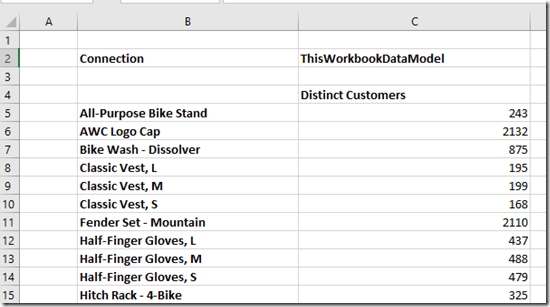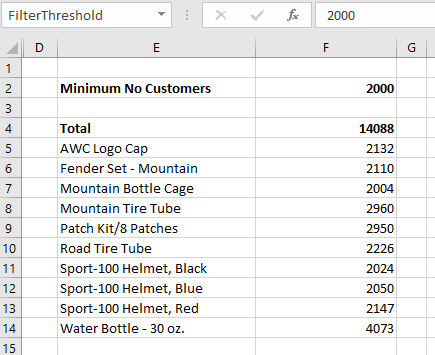# Excel Dynamic Arrays And The CubeSet Function

Among all the exciting announcements made at Ignite last week, one you may have missed (even in all the Excel-related announcements here) was that dynamic arrays have finally reached GA. Ever since they were announced I’ve been interested in how they can be used with Excel cube functions, which allow you to get data from Analysis Services, Power BI and the Excel Data Model/Power Pivot into cells on the worksheet, and I’ve blogged about this once already. Even though right now the CubeValue function can’t be used with dynamic arrays – alas – there are still some interesting ways dynamic arrays and cube functions can be used together and in this post I’ll show you another one I’ve found.

Say you have the following set of tables in the Excel Data Model:And the following measure that counts the number of customers who bought something:

```Distinct Customers:=DISTINCTCOUNT('Internet Sales'[CustomerKey])

```

Let’s also say you have on a worksheet the following table of data showing the number of distinct customers broken down by product, created using the CubeMember and CubeValue functions:Here are the formulas for this table:So far, all very straightforward. Now let’s say you want to use a dynamic array to filter the rows in this table so you only see the products that have more than a certain number of distinct customers, and you want to see a grand total for this filtered list of customers, like so:Here are the formulas to achieve this:To explain what’s going on here:

• Cell C2 contains the text “ThisWorkbookDataModel”, the name of the connection to the Excel Data Model. Doing this makes the other Excel formulas here much easier to read!
• Cell F2 – which I’ve turned into a named range called FilterThreshold – contains the number of customers which a product must exceed to be shown.
• Cell E5 contains the dynamic array Filter function that filters the rows shown in the first two screenshots above and only returns the products that exceed the threshold. The formula is:
FILTER(B5:B134,C5:C134>FilterThreshold)
• Cell E6 does something similar to E5 but returns the number of distinct customers for each product. If all I wanted was the products and the number of distinct customers I wouldn’t have needed two separate formulas, I could have used one, but I need to add the grand total too…
• Cell E4 contains the formula:
CUBESET(\$C\$2, E5#, “Total”)
This creates a set from the spill reference E5#, which contains the cells containing CubeMember formulas returned by the Filter function. For some reason CubeSet returns an error if you try to use a dynamic array formula in its second parameter but using a spill reference works ok; this is why I had to use separate formulas in E5 and E6.
• Finally, cell F4 contains the formula that returns the total distinct count for all products returned in the filtered list using CubeValue and the set created in cell E4:
CUBEVALUE(\$C\$2, “[Measures].[Distinct Customers]”, E4)
Since this is a distinct count the only way to get the correct value here is using CubeSet, CubeValue and the DAX measure – there would be no way to calculate the correct value using Excel formulas. The same could be said of almost any other DAX measure that wasn’t a simple aggregation.

1.m-b says: